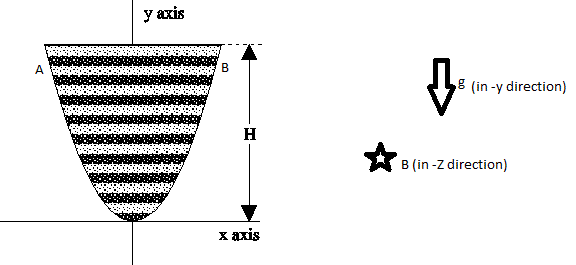# Falling and Shrinking RodLet an Hypothetical thin conducting rod AB is sliding without friction on a parabolic Path: $y={ x }^{ 2 }$. in the X-Y plane from hight 'H' from vertex of parabola and where gravity also exist in -Y direction.

Also an uniform magnetic field exist in -Z direction. Such that it's Magnitude is 'B' . Now conducting Rod is released under gravity at time t=0.

This conducting rod has Special Property That it always remains just inside the Parabola. Which means its length reduced continuously to just fit in Parabola. In this Process linear mass density $'\lambda '$. of rod remains constant.

Then find the induced emf developed across the ends of conducting Rod at the instant when it reaches at hight$\frac { H }{ 2 }$ , from vertex of Parabola.

Assumptions

$\bullet$ When mass is continuously detached from rod with time then there is no extra impulse act's on rod.

$\bullet$ Conducting Rod Has Infinitely Large Resistance.

$\bullet$ No friction between rod and Parabola.

$\bullet$ In this Process linear mass density $'\lambda '$. of rod remains constant.

$\bullet$ Air resistance is negligible.

$\bullet$ Dark lines shown in figure is the conducting Rod at different different time.

$\bullet$ This is An Hypothetical Rod not Found on earth.

Details

$\bullet \quad B=0.5\quad T\\ \bullet \quad H=2\quad m\\ \bullet \quad g=10\quad m/{ s }^{ 2 }$.

###### This is Part of set mixing of concepts
×

Problem Loading...

Note Loading...

Set Loading...